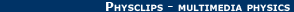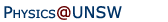Relativity in brief... or in detail..

## Galilean relativity and Newtonian mechanics: an introduction

• Galilean relativity
• Newton's laws of motion
• Newton's third law and the anthropic principle

### Galilean relativity

In his Dialogue Concerning the Two Chief World Systems, Galileo's three characters spend much of the second day discussing relative motion, including whether or not the Earth is stationary. Before the invention of trains, a ship was perhaps the best example of a frame of reference moving smoothly with respect to the Earth, and so Galileo chooses this example. (The jugglers in our animation are not mentioned, and I have taken some liberties.) In particular, they discuss the dropping of a rock from the top of the mast of a smoothly moving ship. In such an experiment, the rock falls (almost exactly) at a point on the deck vertically below where the mast head is at the time the ball lands. Note that it does not land at a point well behind the mast, a point that, as measured by somebody on shore, was below the mast head when the rock was dropped. Galileo makes the point that the ancients, presumably meaning Aristotle, described the result of such an experiment without having done it. (How do I know which is correct? Yes, I have done versions of this experiment. In lectures, I tape a container low on the fork of my bicycle, then drop a ball from directly above it, while riding. Also see below.)

Let's look at a situation inspired by Galileo's example. An observer on a train moving smoothly with velocity v either drops a ball or throws it horizontally with speed -v, ie with speed v in the opposite direction. We can compare this with an observer on the platform who either drops a ball, or who throws it (forwards) with v. We show the two points of view. Galileo chose lead or rock as the material so that the weight of the projectile would be much greater than the forces exerted on it by air. In our animation, air resistance is neglected.

What happens on the platform. From our viewpoint, also at rest in the station, we see that the ball dropped by the man at right on the platform falls vertically. The ball thrown horizontally at v by the man at left on the platform follows a parabolic path.

What happens on the train. The train is travelling at v, so when the man at left on the train drops a ball, the observer on the platform sees it follow a parabolic path: if follows the same path (with respect to us) as the ball thrown by the man on the left of the platform. However, the man on the train sees the ball land at his feet: in his frame of reference, the ball he dropped has fallen vertically.

For symmetry, the man at right on the train throws a ball at -v with respect to the train. We see its horizontal speed as v+(-v) = 0: in our frame of reference, it falls vertically, but to him it travels along a parabola.

Of course, anything is possible in an animation. Science relies on experiment and observation. Next time you are on a train, stand still and drop a small dense object (a coin would do) on the floor. Does it land near your feet, or does it land where your feet were when you released it? Depending on how tall you are and how you hold it, it will take about half a second to fall. If the train travels at say 100 k.p.h., your feet will have travelled about 15 metres during the fall, so the results of the experiment are likely to be clear. (For safety reasons, do not drop or throw objects outside a train (where in any case air resistance will often be significant). However, you and a friend might like to try experiments using a bicycle as described above.)

You might also care to conduct an experiment when travelling in an aircraft. Sitting in your seat, you drop a fork into your lap. You and the fork are both travelling at several hundred kilometres per hour, with respect to the ground. It takes the fork a quarter of a second to fall the first 30 cm. During this time, the plane (and you, and the fork) travel 40 metres. I suggest doing this experiment only because I am quite confident that the fork will fall in your lap: it will not collide with your chest at hundreds of kilometres per hour. For this experiment, only the relative motion is important.

Galileo's work reported in Dialogue Concerning the Two Chief World Systems actually makes a lot of progress towards what we now know as Newtonian mechanics, which is our next topic.

### Newton's laws

Newtonian mechanics, including the aspects developed by Galileo and others, was at least as revolutionary as Einstein's theory. In the West and North Africa at least, ideas about mechanics had previously been dominated by the writings of Aristotle.

One of the big differences was this: for Aristotle, the 'natural' state of matter was at rest (with respect to the Earth). For Galileo and Newton, the 'natural' state of matter could be said to be constant velocity. For Aristotle, there is no need to ask why common objects come to a stop: that is their natural state. Rather, there is a need to ask why some objects (e.g. planets) keep moving. Now look at Newton's laws. The italic is a translation of Newton's own words, the roman text is my paraphrasing.

Newton's first law: Every body perseveres in its state of rest, or of uniform motion in a right line, unless it is compelled to change that state by forces impressed thereon. If no force acts on a body, then it travels in a straight line at constant speed. i.e. its velocity is constant and its acceleration is zero.

Newton's second law: The alteration of motion is ever proportional to the motive force impressed; and is made in the direction of the right line in which that force is impressed. (using bold for vectors, see below) F = ma. Or, in words: a net force F accelerates a body in the direction of the force. To any body may be ascribed a scalar constant, its mass m, such that the acceleration a produced in two bodies by a given force is inversely proportional to their masses, ie, for a given F, a2/a1 = m1/m2.

Newton's third law: To every action there is always opposed an equal and opposite reaction: or the mutual actions of two bodies upon each other are always equal, and directed to contrary parts. Forces always come in pairs, and the sum of the pair is zero.

Newton's laws as equations
When we look at forces specifically (as we do later for electricity and magnetism), we always define forces in pairs. There is an electrical attraction between an electron and a proton, and the force on the electron due to the proton has the same magnitude as that on the proton due to the electron but is in the opposite direction. The gravitational force the earth exerts on the moon has the same magnitude as that exerted by the moon on the earth. Or in general, for bodies 1 and 2, and for any interaction between them,

Newton's third law:    F1,2 = -F2,1.
If we write the total force acting a body as F, its mass as m and its acceleration as a, then the we may write
Newton's first and second law combined:    F = ma.
For a body of finite size, one might ask what acceleration is meant. In this case, a is the acceleration of its centre of mass. We might also ask what forces are included. Here, the third law is helpful. All of the internal forces come in pairs that add to zero, so we need consider only the external forces.

### Newton's third law and the anthropic principle

Usually, scientific laws are developed by induction from experiment. They cannot be proved. For that reason, the work-energy theorem (the kinetic energy ½mv2 of a point mass is increased by an amount equal to the total work done on it) is not a law: it is a theorem, a logical consequence of Newton's second law and some simple calculus. However, there is an indirect 'explanation', if not a proof, of Newton's third law.

Consider an object (make the example concrete: say a pen). According to Newton's third law, the force exerted by the bottom half of the pen on the top half plus that exerted by the top on the bottom add to zero. Now imagine that the third law is false, and that these two forces together produce a non-zero force on the pen. So it accelerates, and continues to do so. A universe in which objects spontaneously accelerated to large relative velocities would be a very dangerous place to live, mammals would not have evolved, and one of them would not be sitting here writing obscure footnotes. This is an example of the weak anthropic principle: if you find that the universe has laws and/or conditions that allow the existence of a being such as yourself, then you should not be surprised. There is also a strong anthropic principle, which surfaces from time to time in science, and with which I have some fun in this link.

We give a more complete introduction to Newton's laws in Physclips: Mechanics with animations and film clips.

•Home | Summary | Quiz | Credits School of Physics - The University of New South Wales Sydney, Australia 2052 © School of Physics UNSW 2052 Disclaimer feedback